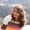FREELessons: 20Length: 1.7 hours• Overview
• Transcript

# 3.5 Loops and Iterators: the for Loop

In this lesson, I'll explain how to write loops and iterators in Kotlin. I'll show you how to use the for loop with an example.

## 5.Conclusion1 lesson, 01:17

### 3.5 Loops and Iterators: the for Loop

Hello there. We have covered a lot of topics regarding the syntaxes and basics of Kotlin. In this lesson, we will be learning about the loops and iterators. Basically, there are three loops which are widely used. They are named as the FOR loop, WHILE loop, and the DO WHILE loop. The three conventional loops that are present in all the programming languages, including Java. So we will begin with the very basics here. If we want to print Hello World for a single time, we write printIn("Hello World") and then we run our code. And here we get the output as Hello World. But what if you want to execute this line of code five times? You write printIn("Hello World") five times, and then we execute our code. And we get Hello World written five times. But this is not the programming way to do this. If we are want to execute any code typically, we need to use loops and iterators. That is what loops and iterators are for. So we will first use the FOR loop to execute this line of code for let's say five times. We will use the ranges and dot operators here. If you haven't already seen it, please refer to the lesson which talks about ranges and dot operators. Because we will be using it throughout this lesson. So beginning with the for loop, the syntax is somewhat like this. Redefine a counter variable, which says (in 1..5) and then we have this statement which has to be executed inside the loop. So for the loops in Kotlin, we have three things here. First is i, which is the counter variable. The second is the range, which will be used for checking the condition, or we can see the condition verification. And the third is incrementing or decrementing the account variable. So what basically happens here in this line of code is, it checks the counter variable that is i. In, in is the operator that we use to verify the condition that i is present within the range of 1 to 5. I will be first assigned as 1, the value of i is automatically increased and hence the loop continues till the value of i is 5. Let us print i here so that it will be convenient for you to see how it works. Now let us run the code and see what happens. So here we are. We have one Hello World, two Hello World, three, four, five Hello World. So this line of code is executed when this condition is true. And once this condition is false, it comes out of the loop, and the execution stops. So this was all about the FOR loop. In the next lesson we will be learning about the WHILE, and the DO WHILE loop. That's all for this lesson, keep smiling, and stay tuned.

Back to the top